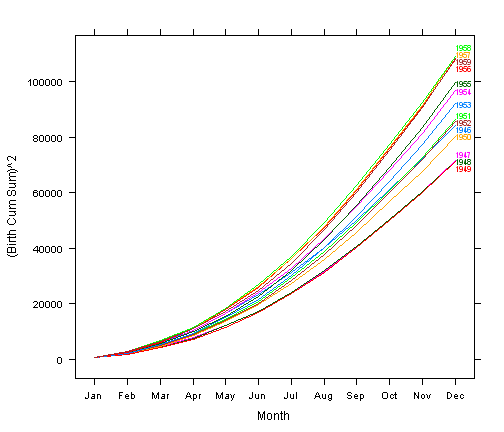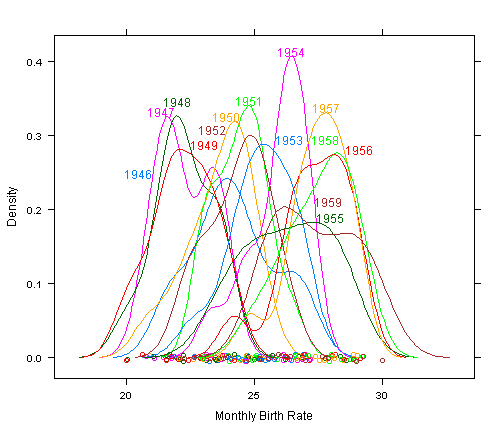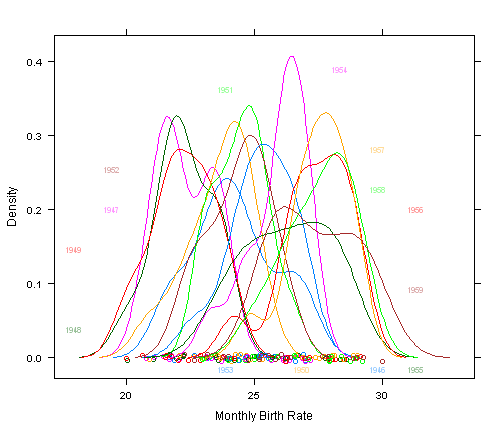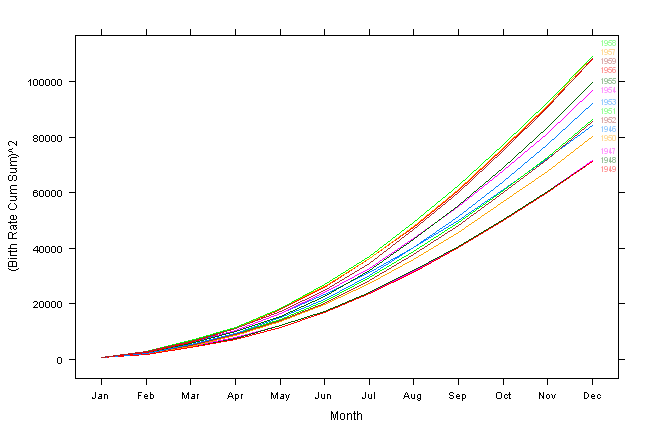## Thursday, 12 December 2013

### Insert Labels in the Graph - 'directlabels' Package

In general, a legend is used to display categorical values used as groups or keys in a graph. In those cases, different colours or different shapes/formats are used to identify the points or lines in the graph that corresponds to the described keys in the legend. This has a limitation when there is a large number of keys to display. In such cases, it is more effective to insert the actual keys adjacent to the corresponding points or lines in the graph.

To insert labels inside the graph, text() function is usually used. This method is described in my other blog post titled Supplementary functions for plot(). As it can be seen from this blog post, it is rather cumbersome. 'directlabels' package makes this task much simpler if you use ggplot2 or lattice plots.

As shown in the below example, all it requires to insert label in the graph is to wrap the plotting function with direct.label() function. The data used in the example was obtained from http://robjhyndman.com/tsdldata/data/, and the name of the data set is nybirths.dat. It contains records of number of birth in New York on a monthly basis between 1946 and 1959.

library(directlabels)

births <- scan("http://robjhyndman.com/tsdldata/data/nybirths.dat") my <- seq(from = as.Date("01-Jan-1946", format = "%d-%b-%Y"), by = "months", length = 168)

This will calculate cumulative sums.

cs <- matrix(births, 12, 14) cs <- apply(cs, 2, cumsum) births_cs <- data.frame(Year = format(my, "%Y"), Month = format(my, "%b"), birth_rate = births, Birth_cs = as.vector(cs))

In this graph, x-axis represents months, but if the months are written in alphabets, R has a tendency to sort them in alphabetical order. Hence, we need to sort this in line with the numeric order, i.e. in time series mode, by doing below.

births_cs\$Month <- factor(births_cs\$Month, levels = format(my[1:12], "%b"))

In this graph, I deliberately used squared values of the cumulative sum on the y-axis, as this will show the yearly differences more clearly. The observation in the graph shows relatively higher birth rate between 1956 and 1959 which coincides with the period of rapid development in New York following the end of World War II (source: http://en.wikipedia.org/wiki/History_of_New_York_City_(1946%E2%80%9377)).

direct.label(xyplot((Birth_cs)^2 ~ Month, births_cs, group = births_cs\$Year, type = "l", xlab = "Month", ylab = "(Birth Cum Sum)^2"))The below is a density plot showing monthly birth rates by year. It shows consistently higher birth rates for 1950's, especially late 1950's, compared to 1940's. Something odd is that birth rates in 1946 is higher than other years in 1940's, almost as high as the rates in 1950's. This may be the baby boom after the war, while the high birth rates in 1950's was contributed by improved economic situation.

direct.label(densityplot(~birth_rate, births_cs, group = births_cs\$Year, xlab = "Monthly Birth Rate", ylab = "Density", n = 100))When there is a large number of lines intertwined as is the case in the above graph, the inserted labels may not effectively align itself to the lines they correspond to. direct.label() contains parameters that allow users to have some control over the transparency and position of the labels.

direct.label(densityplot(~birth_rate, births_cs, group = births_cs\$Year, xlab = "Monthly Birth Rate", ylab = "Density", n = 100), list("chull.grid", cex = 0.7, alpha = 0.4))The control parameters provided by direct.label() is fairly comprehensive with different options that would suit different types of graph. Complete list of all available options can be found here

The below example shows ways of adjusting the label positions vertically (vjust) and horizontally (hjust).

direct.label(xyplot((Birth_cs)^2 ~ Month, births_cs, group = births_cs\$Year, type = "l", xlab = "Month", ylab = "(Birth Rate Cum Sum)^2"), list("last.qp", cex = 0.7, alpha = 0.4, vjust = 0.2, hjust = (-0.5)))